[This article was first published on Avulsos by Penz - Articles tagged as R , and kindly contributed to R-bloggers]. (You can report issue about the content on this page here)
Want to share your content on R-bloggers? click here if you have a blog, or here if you don't.

On the second article, we saw how to use a Monte
Carlo simulation generate sample of disk space delta for future dates and
calculate the distribution probability of zeroing free space in the future.

In this article, we will see how we can plot the evolution of predicted
distribution for the occupied disk space. Instead of answering que question “how
likely is that my disk space will zero before date X?,” we will answer
“how much disk space will I need by date X, and with what probability?”

# The input data

This file has the dataset we will use as example. It’s
the same we used in the second part. The graph below shows it:We now import this data into R:

```
colClasses=c("Date","numeric"),
col.names=c("day","usd"))
attach(duinfo)
totalspace <- 450000
today <- tail(day, 1)

```

We then build our simulations for the next 4 months:

```  # Number of Monte Carlo samples
numsimulations <- 10000

# Number of days to simulate
numdays    <- 240

# Simulate:
simulate <- function(data, ndays) {
delta <- diff(data)
dssimtmp0 <- replicate(numsimulations, tail(data, 1))
dssimtmp  <- dssimtmp0
f <- function(i) dssimtmp <<- dssimtmp + replicate(numsimulations, sample(delta, 1, replace=TRUE))
cbind(dssimtmp0, mapply(f, seq(1, ndays)))
}
dssim <- simulate(usd, numdays)

# Future days:
fday <- seq(today, today+numdays, by='day')

```

# Visualizing the possible scenarios

What king of data have we built in our simulations? Each simulation is
built by sampling from the delta samples and adding to the current disk space
for each day in the simulated period. We can say that each individual simulation
is a possible scenario for the next 4 months. The graph bellow shows the
first 5 simulations:

```  plot(fday, dssim[1,], ylim=c(min(dssim[1:5,]), max(dssim[1:5,])), ylab='usd', xlab='day', xaxt='n', type='l')
axis.Date(1, day, at=seq(min(fday), max(fday), 'week'), format='%F')
lines(fday, dssim[2,])
lines(fday, dssim[3,])
lines(fday, dssim[4,])
lines(fday, dssim[5,])
abline(h=totalspace, col='gray')
```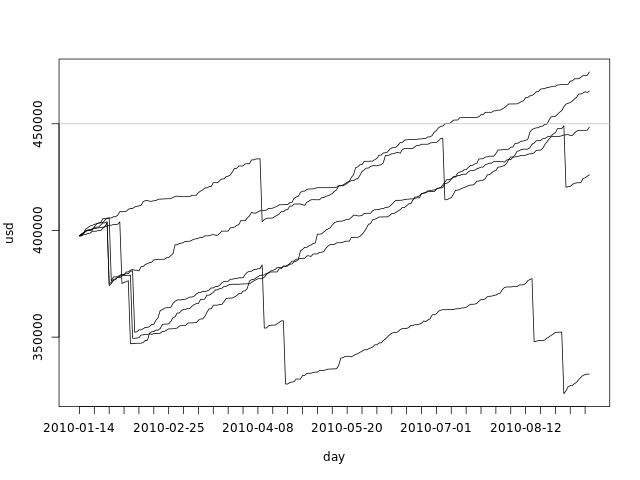From this graph we can clearly see that the range of possible values for the
used disk space grows with time. All simulations start with the same value - the
used disk space for today - and grow apart as we sample from the delta pool.

We can also plot all simulations in a single graph:

```  plot(fday, dssim[1,], ylim=c(min(dssim), max(dssim)), ylab='usd', xlab='', xaxt='n', type='l')
axis.Date(1, day, at=seq(min(fday), max(fday), 'week'), format='%F')
f <- function(i) lines(fday, dssim[i,])
mapply(f, seq(2, numdays))
abline(h=totalspace, col='gray')
```This plot gives us an idea of the overall spread of the data, but it fails to
show the density. There are 10000 black lines there, with many of them
overlapping one another.

# Visualizing the distribution for specific days

There is another way to look at our data: we have created, for each day, a
sample of the possible used disk spaces. We can take any day of the simulation
and look at the density:

```  dssimchosen <- list(density(dssim[,5]), density(dssim[,15]), density(dssim[,45]), density(dssim[,120]))
colors <- rainbow(length(dssimchosen))
xs <- c(mapply(function(d) d\$x, dssimchosen))
ys <- c(mapply(function(d) d\$y, dssimchosen))
plot(dssimchosen[], xlab='usd', ylab='dens',
xlim=c(min(xs),max(xs)), ylim=c(min(ys),max(ys)), col=colors, main='')
lines(dssimchosen[], col=colors)
lines(dssimchosen[], col=colors)
lines(dssimchosen[], col=colors)
abline(v=totalspace, col='gray')
legend('top', c('5 day', '15 days', '45 days', '120 days'), fill=colors)
```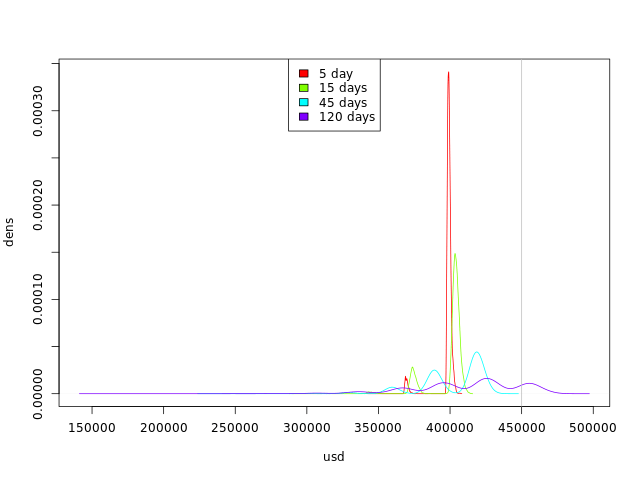By looking at this graph we can see the trend:

• The curves are getting flatter: we are getting more possible values for
occupied disk space.
• The curves are moving to the right: we have more simulations with higher
occupied disk space values.

# Visualizing the evolution of the distribution

So far, we have seen how we can visualize some simulations along the 4 months
and how we can visualize the distribution for some specific days.

We can also plot the distribution of the values for each day in the simulated 4
months. We can't use the kernel density plot or the histogram, as they use both
axes, but there are other options, most of them involving some abuse of the
built-in plot functions.

## Boxplot

We can use the boxplot function to create a boxplot for each day in R in a
very straightforward way:

```  boxplot(dssim, outline=F, names=seq(today, as.Date(today+numdays), by='day'), ylab='usd', xlab='day', xaxt='n')
abline(h=totalspace, col='gray')
```The boxplots glued together form a shape that shows us the distribution of our
simulations at any day:

• The thick line in the middle of the graph is the median
• The darker area goes from the first quartile to the third - which means that
50% of the samples are in that range
• The lighter area has the maximum and minimum points, if they are within 1.5
IQR of the upper/lower
quartile. Points out of this range are considered outliers and are not
plotted.

## Quantile lines

We can use the quantile function to calculate the values of each
quantile per day, and plot the lines:

```  q <- 6
f <- function(i) quantile(dssim[,i], seq(0, 1, 1.0/q))
qvals <- mapply(f, seq(1, numdays+1))
colors <- colorsDouble(rainbow, q+1)
plot(fday, qvals[1,], ylab='usd', xlab='day', xaxt='n', type='l', col=colors, ylim=c(min(qvals), max(qvals)))
mapply(function(i) lines(fday, qvals[i,], col=colors[i]), seq(2, q+1))
axis.Date(1, day, at=seq(min(fday), max(fday), 'week'), format='%F')
abline(h=totalspace, col='gray')
```The advantage of this type of graph over the boxplot is that it is parameterized
by q. This variable tells us the number of parts that we should divide our
sample in. The lines above show us the division. If q is odd, the middle
line is exactly the median. If q is 4, the lines will draw a shape similar
to that of the boxplot, the only difference being the top and bottom line, that
will include outliers - the boxplot filters outliers by using the IQR as
explained above.

In the code above, we have used the colorsDouble function to generate a
sequence of colors that folds in the middle:

```  colorsDouble <- function(colorfunc, numcolors) {
colors0 <- rev(colorfunc((1+numcolors)/2))
c(colors0, rev(if (numcolors %% 2 == 0) colors0 else head(colors0, -1)))
}
```

## Quantile areas

We can also abuse the barplots function to create an area graph. We have to
eliminate the bar borders, zero the distance between them and plot a white bar
from the axis to the first quartile, if appropriate:

```  q <- 7
f <- function(i) {
qa <- quantile(dssim[,i], seq(0, 1, 1.0/q))
c(qa, diff(qa))
}
qvals <- mapply(f, seq(1, numdays+1))
colors <- c('white', colorsDouble(rainbow, q))
barplot(qvals, ylab='usd', xlab='day', col=colors, border=NA, space=0,
names.arg=seq(min(fday), max(fday), 'day'), ylim=c(min(dssim), max(dssim)))
abline(h=totalspace, col='gray')
```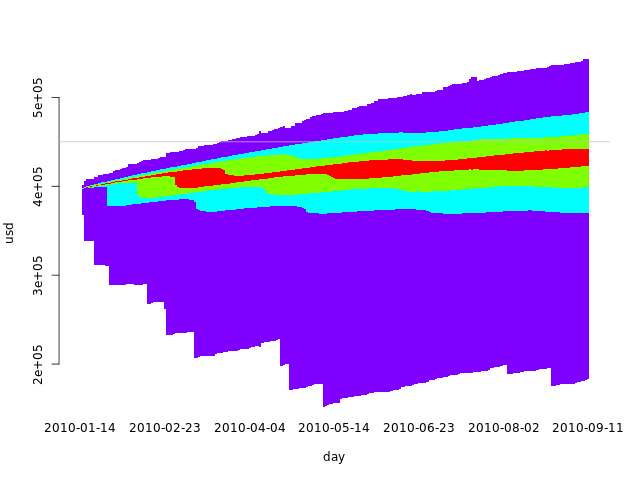In this case, using an odd q makes more sense, as we want to use the same
colors for the symmetric intervals. With an even q, there would either be a
larger middle interval with two quantiles or a broken symmetry. The code above
builds a larger middle interval when given an even q.

## Quantile heat map

If we increase q and use heat.colors in a quantile area plot, we get
something similar to a heat map:

```  q <- 25
f <- function(i) {
qa <- quantile(dssim[,i], seq(0, 1, 1.0/q))
c(qa, mapply(function(j) qa[j] - qa[j-1], seq(2, q+1)))
}
qvals <- mapply(f, seq(1, numdays+1))
colors <- c('white', colorsDouble(heat.colors, q))
barplot(qvals, ylab='usd', xlab='day', col=colors, border=NA, space=0,
names.arg=seq(min(fday), max(fday), 'day'), ylim=c(min(dssim), max(dssim)))
abline(h=totalspace, col='gray')
```# Visualizing past, present and future

We can also plot our data in the same graph as our simulations, by extending the
axis of the barplot and using the points function:

```  quantheatplot <- function(x, sim, ylim) {
q <- 25
simstart <- length(x) - length(sim[1,])
f <- function(i) {
if (i < simstart)
replicate(q+1, 0)
else {
qa <- quantile(sim[,i-simstart], seq(0, 1, 1.0/q))
c(qa, diff(qa))
}
}
qvals <- mapply(f, seq(1, length(x)))
colors <- c('white', colorsDouble(heat.colors, q))
barplot(qvals, ylab='usd', xlab='day', col=colors, border=NA, space=0,
names.arg=x, ylim=ylim)
abline(h=totalspace, col='gray')
}

quantheatplot(c(day, seq(min(fday), max(fday), 'day')), dssim, ylim=c(min(c(usd, dssim)), max(dssim)))
points(usd)
abline(h=totalspace, col='gray')
```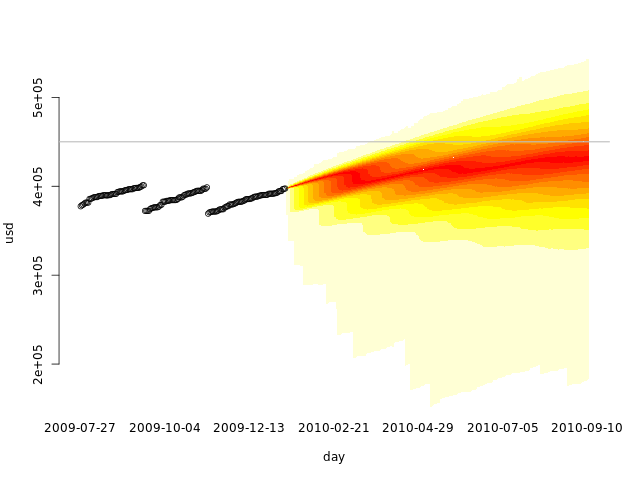# Training set, validation set

Cross-validation is
a technique that we can use to validate the use of Monte Carlo on our data.

We first split our data in two sets: the training set and the validation set. We
than use only the first in our simulations, and plot the second over. We can
then see graphically if the data fits our simulation.

Let's use the first two months as the training set, and the other three months
as the validation set:

```  # Number of days to use in the training set
numdaysTrain <- 60
numdaysVal   <- length(day) - numdaysTrain

dssim2 <- simulate(usd[seq(1, numdaysTrain)], numdaysVal-1)

allvals <- c(usd, dssim2)
quantheatplot(day, dssim2, c(min(allvals), max(allvals)))
points(usd)
```Looks like using only the first two months already gives us a fair simulation.
What if we used only a single month, when no disk cleanup was performed?

```  # Number of days to use in the training set
numdaysTrain <- 30
numdaysVal   <- length(day) - numdaysTrain

dssim3 <- simulate(usd[seq(1, numdaysTrain)], numdaysVal-1)

allvals <- c(usd, dssim3)
quantheatplot(day, dssim3, c(min(allvals), max(allvals)))
points(usd)
```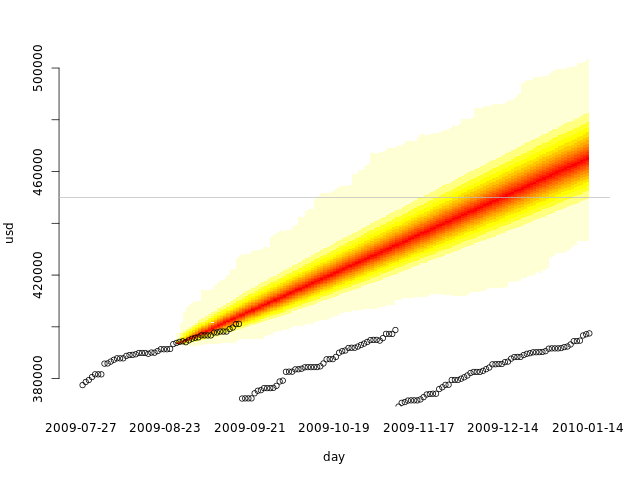If we do regular disk cleanups, we must have at least one of them in our
training set to get realistic results. Our training set is not representative
without it.

This also tests our cross-validation code. A common mistake is using the
whole data set as the training set and as the validation set. That is not
cross-validation.

# Conclusions

We can use Monte Carlo simulations not only to generate a distribution
probability of an event as we did in the previous
article, but also to predict a possible range of future values. In this article,
disk space occupation is not the most interesting example, as we are usually
more interested in knowing when our used disk space will reach a certain value
than in knowing the most probable values in time. But imagine that the data
represents the number of miles traveled in a road trip or race. You can then not
only see when you will arrive at your destination, but also the region where you
will probably be at any day.

There are plenty of other uses for this kind of prediction. Collect the data,
look at it and think if it would be useful to predict future ranges, and if it
makes sense with the data you have. Predictions based on the evidence can be
even used to support a decision or a point of view, just keep mind that
you can only use the past if you honestly don't think anything different is
going to happen.